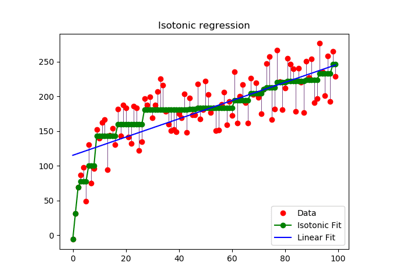/scikit-learn

# sklearn.isotonic.IsotonicRegression

`class sklearn.isotonic.IsotonicRegression(y_min=None, y_max=None, increasing=True, out_of_bounds=’nan’)` [source]

Isotonic regression model.

The isotonic regression optimization problem is defined by:

```min sum w_i (y[i] - y_[i]) ** 2

subject to y_[i] <= y_[j] whenever X[i] <= X[j]
and min(y_) = y_min, max(y_) = y_max
```
where:
• `y[i]` are inputs (real numbers)
• `y_[i]` are fitted
• `X` specifies the order. If `X` is non-decreasing then `y_` is non-decreasing.
• `w[i]` are optional strictly positive weights (default to 1.0)

Read more in the User Guide.

Parameters: `y_min : optional, default: None` If not None, set the lowest value of the fit to y_min. `y_max : optional, default: None` If not None, set the highest value of the fit to y_max. `increasing : boolean or string, optional, default: True` If boolean, whether or not to fit the isotonic regression with y increasing or decreasing. The string value “auto” determines whether y should increase or decrease based on the Spearman correlation estimate’s sign. `out_of_bounds : string, optional, default: “nan”` The `out_of_bounds` parameter handles how x-values outside of the training domain are handled. When set to “nan”, predicted y-values will be NaN. When set to “clip”, predicted y-values will be set to the value corresponding to the nearest train interval endpoint. When set to “raise”, allow `interp1d` to throw ValueError. `X_min_ : float` Minimum value of input array `X_` for left bound. `X_max_ : float` Maximum value of input array `X_` for right bound. `f_ : function` The stepwise interpolating function that covers the input domain `X`.

#### Notes

Ties are broken using the secondary method from Leeuw, 1977.

#### References

Isotonic Median Regression: A Linear Programming Approach Nilotpal Chakravarti Mathematics of Operations Research Vol. 14, No. 2 (May, 1989), pp. 303-308

Isotone Optimization in R : Pool-Adjacent-Violators Algorithm (PAVA) and Active Set Methods Leeuw, Hornik, Mair Journal of Statistical Software 2009

Correctness of Kruskal’s algorithms for monotone regression with ties Leeuw, Psychometrica, 1977

#### Methods

 `fit`(X, y[, sample_weight]) Fit the model using X, y as training data. `fit_transform`(X[, y]) Fit to data, then transform it. `get_params`([deep]) Get parameters for this estimator. `predict`(T) Predict new data by linear interpolation. `score`(X, y[, sample_weight]) Returns the coefficient of determination R^2 of the prediction. `set_params`(**params) Set the parameters of this estimator. `transform`(T) Transform new data by linear interpolation
`__init__(y_min=None, y_max=None, increasing=True, out_of_bounds=’nan’)` [source]
`fit(X, y, sample_weight=None)` [source]

Fit the model using X, y as training data.

Parameters: `X : array-like, shape=(n_samples,)` Training data. `y : array-like, shape=(n_samples,)` Training target. `sample_weight : array-like, shape=(n_samples,), optional, default: None` Weights. If set to None, all weights will be set to 1 (equal weights). `self : object` Returns an instance of self.

#### Notes

X is stored for future use, as `transform` needs X to interpolate new input data.

`fit_transform(X, y=None, **fit_params)` [source]

Fit to data, then transform it.

Fits transformer to X and y with optional parameters fit_params and returns a transformed version of X.

Parameters: `X : numpy array of shape [n_samples, n_features]` Training set. `y : numpy array of shape [n_samples]` Target values. `X_new : numpy array of shape [n_samples, n_features_new]` Transformed array.
`get_params(deep=True)` [source]

Get parameters for this estimator.

Parameters: `deep : boolean, optional` If True, will return the parameters for this estimator and contained subobjects that are estimators. `params : mapping of string to any` Parameter names mapped to their values.
`predict(T)` [source]

Predict new data by linear interpolation.

Parameters: `T : array-like, shape=(n_samples,)` Data to transform. `T_ : array, shape=(n_samples,)` Transformed data.
`score(X, y, sample_weight=None)` [source]

Returns the coefficient of determination R^2 of the prediction.

The coefficient R^2 is defined as (1 - u/v), where u is the residual sum of squares ((y_true - y_pred) ** 2).sum() and v is the total sum of squares ((y_true - y_true.mean()) ** 2).sum(). The best possible score is 1.0 and it can be negative (because the model can be arbitrarily worse). A constant model that always predicts the expected value of y, disregarding the input features, would get a R^2 score of 0.0.

Parameters: `X : array-like, shape = (n_samples, n_features)` Test samples. For some estimators this may be a precomputed kernel matrix instead, shape = (n_samples, n_samples_fitted], where n_samples_fitted is the number of samples used in the fitting for the estimator. `y : array-like, shape = (n_samples) or (n_samples, n_outputs)` True values for X. `sample_weight : array-like, shape = [n_samples], optional` Sample weights. `score : float` R^2 of self.predict(X) wrt. y.
`set_params(**params)` [source]

Set the parameters of this estimator.

The method works on simple estimators as well as on nested objects (such as pipelines). The latter have parameters of the form `<component>__<parameter>` so that it’s possible to update each component of a nested object.

Returns: self
`transform(T)` [source]

Transform new data by linear interpolation

Parameters: `T : array-like, shape=(n_samples,)` Data to transform. `T_ : array, shape=(n_samples,)` The transformed data

## Examples using `sklearn.isotonic.IsotonicRegression`Isotonic Regression

© 2007–2018 The scikit-learn developers
Licensed under the 3-clause BSD License.
http://scikit-learn.org/stable/modules/generated/sklearn.isotonic.IsotonicRegression.html